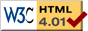Nav: [home] > [fun] > [proofs]

## Fun: Mathematical Proofs

### Proof: Women are Evil

It is well-known theorem that

time = money

We also know that money is the root of all evil, or expressed mathematically:

money = sqrt(evil)

Furthermore, we know that the number of women you get is basically your amount of time multiplied with your amount of money:

women = time * money

Putting the first equation into the third one leads to

women = money2

and by application of the second equation (money2=evil) we find that

women = evil

In other words: Women are evil. Q.E.D.

### Proof: The less you know the more you earn

Two important facts of life are

time = money

knowledge = power

On the other hand, power is work divided by time,

power = work / time

Putting the first two equations into the third one and solving the result for money, we find that

money = work / knowledge

This tells us two things you always knew: The more you work, the more money you get and, more importantly, the less you know the more earn! Q.E.D.